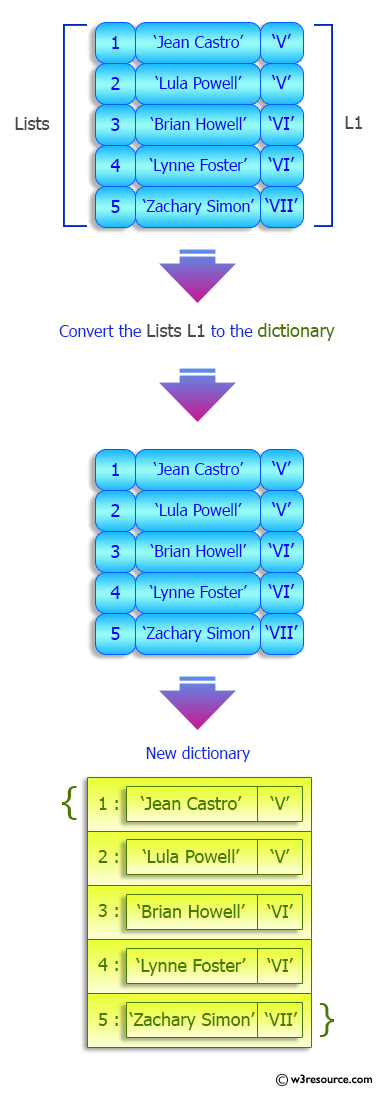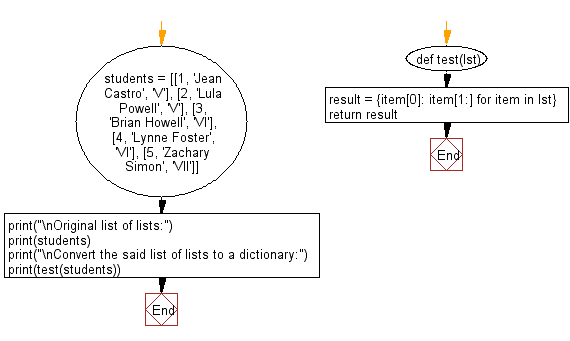﻿ Python: Convert a given list of lists to a dictionary - w3resource# Python: Convert a given list of lists to a dictionary

## Python dictionary: Exercise-63 with Solution

Write a Python program to convert a given list of lists to a dictionary.

Sample Solution:

Python Code:

``````def test(lst):
result = {item: item[1:] for item in lst}
return result

students = [[1, 'Jean Castro', 'V'], [2, 'Lula Powell', 'V'], [3, 'Brian Howell', 'VI'], [4, 'Lynne Foster', 'VI'], [5, 'Zachary Simon', 'VII']]

print("\nOriginal list of lists:")
print(students)
print("\nConvert the said list of lists to a dictionary:")
print(test(students))
```
```

Sample Output:

```Original list of lists:
[[1, 'Jean Castro', 'V'], [2, 'Lula Powell', 'V'], [3, 'Brian Howell', 'VI'], [4, 'Lynne Foster', 'VI'], [5, 'Zachary Simon', 'VII']]

Convert the said list of lists to a dictionary:
{1: ['Jean Castro', 'V'], 2: ['Lula Powell', 'V'], 3: ['Brian Howell', 'VI'], 4: ['Lynne Foster', 'VI'], 5: ['Zachary Simon', 'VII']}
```

Pictorial Presentation:Flowchart:## Visualize Python code execution:

The following tool visualize what the computer is doing step-by-step as it executes the said program:

Python Code Editor:

Have another way to solve this solution? Contribute your code (and comments) through Disqus.

What is the difficulty level of this exercise?

Test your Programming skills with w3resource's quiz.

﻿

## Python: Tips of the Day

Clamps num within the inclusive range specified by the boundary values x and y:

Example:

```def tips_clamp_num(num,x,y):
return max(min(num, max(x, y)), min(x, y))
print(tips_clamp_num(2, 4, 6))
print(tips_clamp_num(1, -1, -6))
```

Output:

```4
-1
```